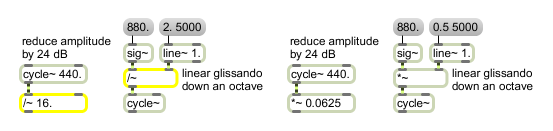# /~

Divide one signal by another

## Description

Note: Division is not a computationally efficient operation. The /~ object is optimized to multiply a signal coming into the left inlet by the reciprocal of either the initial argument or an int or float received in the right inlet. However, when two signals are connected, /~ uses the significantly more inefficient division procedure.

## Arguments

Name Type Opt Description
initial-divisor float or int opt Sets an initial value by which to divide the signal coming into the left inlet. If a signal is connected to the right inlet, the argument is ignored. If no argument is present, and no signal is connected to the right inlet, the initial value is 1 by default.

## Messages

 int dividend/divisor [int] In left inlet: The number is divided by the signal coming into the right inlet. If a signal is also connected to the left inlet, a float or int is ignored. In right inlet: A number by which to divide the signal coming into the left inlet. If a signal is also connected to the right inlet, a float or int is ignored. float dividend/divisor [float] In left inlet: The number is divided by the signal coming into the right inlet. If a signal is also connected to the left inlet, a float or int is ignored. In right inlet: A number by which to divide the signal coming into the left inlet. If a signal is also connected to the right inlet, a float or int is ignored. signal In left inlet: The signal is divided by a signal coming into the right inlet, or a constant value received in the right inlet. In right inlet: The signal is used as the divisor, to be divided into the signal coming into the left inlet, or the constant value received in the left inlet.

## Output

signal: The ratio of the two inputs, i.e., the left input divided by the right input.

## ExamplesIt is more computationally efficient to use an equivalent multiplication when possible